Courses

# Test: Solid Mechanics- 1

## 20 Questions MCQ Test Mock Test Series of SSC JE Civil Engineering | Test: Solid Mechanics- 1

Description
This mock test of Test: Solid Mechanics- 1 for SSC helps you for every SSC entrance exam. This contains 20 Multiple Choice Questions for SSC Test: Solid Mechanics- 1 (mcq) to study with solutions a complete question bank. The solved questions answers in this Test: Solid Mechanics- 1 quiz give you a good mix of easy questions and tough questions. SSC students definitely take this Test: Solid Mechanics- 1 exercise for a better result in the exam. You can find other Test: Solid Mechanics- 1 extra questions, long questions & short questions for SSC on EduRev as well by searching above.
QUESTION: 1

### The maximum positive bending moment in a fixed beam of span 8m and subjected to a central point load of 120 kN is (in kN-m)

Solution:

Bending moment at centre of the span

= (W×L)/4      ie (120 kn × 8 m )/4

= 240 kn-m.

Since the load acting in the middle of span. Hence the load will be equally distributed at both endsTherefore max bending moment in fixed beam

= 240 kn-m / 2= 120 kn-m .

QUESTION: 2

### The major and minor principal stresses at a point are 3Mpa and -3Mpa respectively. The maximum shear stress at the point is

Solution:

Maximum shear stress (τ) is given by: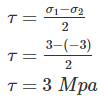QUESTION: 3

### A point within the cross sectional plane of a beam through which the resultant of the external loading on the beam has to pass through to ensure pure bending without twisting of the cross-sectional of the beam is called:

Solution:

Shear centre is a point from which a concentrated load passes then there will be only bending and no twisting. It is also called centre of flexure. It is that point through which the resultant of shear passes. To avoid twisting and only cause bending, it is necessary for the forces to act through the particular point, which may not coincide with the centroid.

QUESTION: 4

A rectangular beam is 24 cm wide and 50 cm deep, its section modulus is given by:

Solution:

Section modulus is defined as the ratio of moment of inertia of a beam about its C.G to the maximum distance of extreme x-section of the beam (Ymax).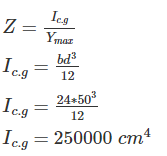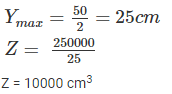QUESTION: 5

The fixed support in a real beam becomes in the conjugate beam as:-

Solution:
QUESTION: 6

The shear stress on a beam section is maximum:-

Solution:
QUESTION: 7

A simple supported beam with rectangular cross section is subjected to a concentrated load at mid span. If the width and depth of beam are doubled, the deflection at mid span will be reduced to:-

Solution:

Max. deflection at mid span(δ)=5WL4/384EI

Hence,deflection is inversely proportional to the moment of inertia.

For rectangular section,I=bd3/12

Therefore,if width and depth both are doubled,deflection will get reduced to 6.25%.

QUESTION: 8

A tensile test is performed on a round bar. After fracture it has been found that the diameter remains approximately same at fracture. The material under test was

Solution:

In Brittle materials under tension test undergoes brittle fracture i.e there failure plane is 90° to the axis of load and there is no elongation in the rod that’s why the diameter remains same before and after the load. Example: Cast Iron, concrete etc

But in case of ductile materials, material first elongate and then fail, their failure plane is 45° to the axis of the load. After failure cup-cone failure is seen. Example Mild steel, high tensile steel etc.

QUESTION: 9

Consider the following statements:

1. On a Principal plane, only normal stress acts

2. On a Principal plane, both normal and shear stresses acts

3. On a Principal plane, only shear stress acts

4. Isotropic state of stress is independent of frame of reference

Which of the above statements is/are correct?

Solution:

Principal planes are the planes at which only normal stress is acting and shear stress is zero at that plane,

Option 2 and 3 are wrong. So, correct option is 1 and 4

QUESTION: 10

The ratio of crippling loads of a column having both the ends fixed to the column having both the ends hinged, is

Solution: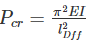For both ends fixed,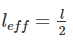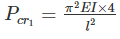For both ends hinged, leff = l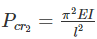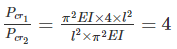QUESTION: 11

What is the limit to Poisson's ratio?

Solution:

Limit of poisons ratio varies between -1 to 0.5.

μ = 0.5 for rubber.

QUESTION: 12

Match the following beams with their bending moment diagrams: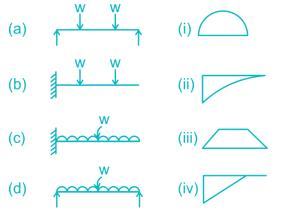Solution:

Bending moment for two point load in simply supported beam will be trapezoidal.

Bending moment diagram for UDL in simply supported beam will be parabolic.

BMD for UDL in cantilever beam as shown in figure (ii)

QUESTION: 13

The crippling load taken by a column with both ends hinged is 100 kN. The crippling load taken by the same column with one end fixed and other end free will be:

Solution:

The crippling load carrying capacity,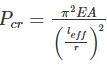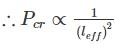if all other parameters remain constant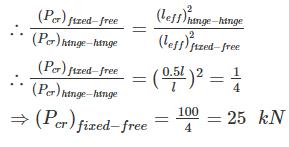QUESTION: 14

Modulus of rigidity is defined as the ratio of ________.

Solution:

The ratio of tensile stress or compressive stress to the corresponding strain is a constant. This ratio is known as Young’s Modulus or Modulus of Elasticity and is denoted by E.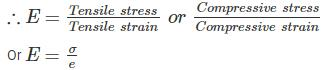The ratio of shear stress to the corresponding shear strain within the elastic limit, is known as Modulus of Rigidity or Shear Modulus. This is denoted by C or G or N.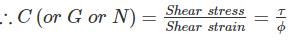QUESTION: 15

The Mohr’s circle given below corresponds to which one of the following stress condition.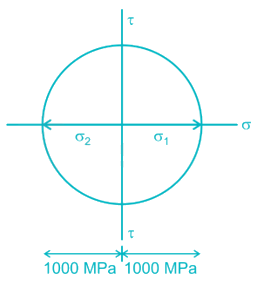Solution:

Radius of Mohr’s circle = 100 MPa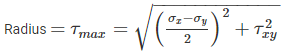And centre of Mohr’s circle is at a distance,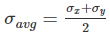from the origin. Here it is in origin.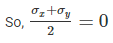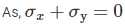both the normal stress may be zero which is a pure shear case or opposite in nature which don’t exist in any of the options. So it is the pure shear case, where the radius is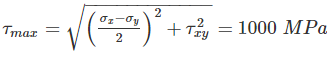QUESTION: 16

If maximum principal stress σ1 = 60 N/mm2, σ2 and σ3 of value zero act on a cube of unit dimensions, then the maximum shear strain energy stored in it would be

Solution:

Shear strain energy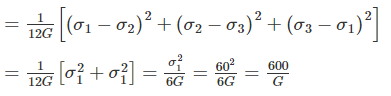QUESTION: 17

If the deflection and slope at the free end of a cantilever beam subjected to the uniformly distributed load are 15 mm and 0.02 radians respectively. The length of the beam is _______.

Solution:

Deflection at the free end of a cantilever beam subjected to udl,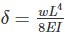Slope at free end subjected to udl,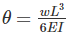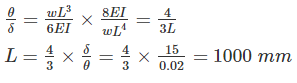QUESTION: 18

The stress in a body due to suddenly applied load compared to when it is applied gradually is _______.

Solution:

here, work done is given as (F δL) / 2 and strain energy stored = (σ2 / 2E) AL

Work done is equal to the strain energy stored.

(F δL) / 2 = (σ2 / 2E) AL

Therefore, σ = (F/A) ----------- (1)

Suddenly applied load is given as σ = (2F/A), here work done = (F δL)

(F δL) = (σ2 / 2E) AL

Therefore, σ = (2F/A) ------------- (2)

From (1) and (2), it can be concluded that

QUESTION: 19

If the deflection at the free end of a cantilever beam of span 1 m, subjected UDL over entire span is 15 mm, then the slope at the free end is ________

Solution:

Deflection at the free end of a cantilever beam subjected to udl,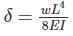Slope at free end subjected to udl,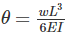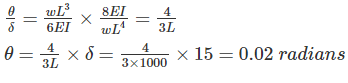QUESTION: 20

For a given material, the modulus of rigidity is 120 GPa and Poisson’s ratio is 0.4. The value of modulus of elasticity in GPa is

Solution:

E = 2G (1 + μ) = 2 × 120 (1 + 0.4) = 336 GPa